Next: Texture Analysis and Synthesis: Up: Introduction Previous: Organisation of the Thesis

# Notational Conventions

This section provides the notational conventions used throughout this thesis.

A digital image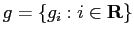on a 2D finite arithmetic lattice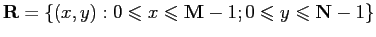is a function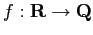that maps the supporting lattice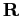onto a finite set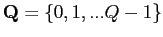of signal values (e.g., grey levels, colours, or multi-band signatures). For simplicity, the set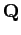is assumed to be integer-valued in the range between 0 and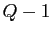.

A pixel in an image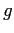is denoted by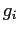where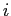is the positioning index in the lattice. The set of all pixels in an imagewith exception of a pixelis denoted by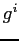. Similarly, all sites on latticeexcept theth site is denoted by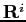, so that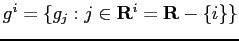.

The notation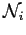defines a neighbourhood of a site, which usually consists of a set of sites with a specific spatial configuration. All sites in a neighbourhood are dependent of each other.

Because the thesis deals mainly with probability texture models, in particular Markov-Gibbs random fields, related terminology and notations are presented below.

A random variable has a nondeterministic value with a given probability distribution. A sequence or array of statistically interrelated random variables form a discrete stochastic process. Let a random variable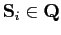be associated with each site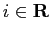. Then these variables form a random field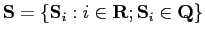in a configuration space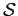if a probability measure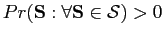exists. A random field is a two-dimensional stochastic process. The configuration space has a combinatorial number of different images,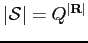.

Under a random field model, an imageis considered as an instance or a sample of the configuration space. The image probability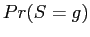, or simply,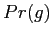, is known as the joint probability of the image signals. Another commonly-used probability measure is conditional probability of a pixelgiven all other pixels, denoted by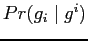. The conditional probability is a local probability of image signals, while the joint probability is a global one.

For convenience's sake, the notational conventions and symbols used in this thesis are summarised in Table 4.1.

 Notation MeaningA 2D finite arithmetic latticeThe set of integral signal valuesA digital image defined onAll pixels in the imageexcept the pixel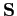A random fieldThe configuration space of a random fieldNeighbourhood of the siteThe latticewith exception of theth site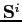All sites ofwith exception of a site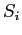Joint probability of a sample imageConditional probability of image signalat pixelgivenNext: Texture Analysis and Synthesis: Up: Introduction Previous: Organisation of the Thesis
dzho002 2006-02-22